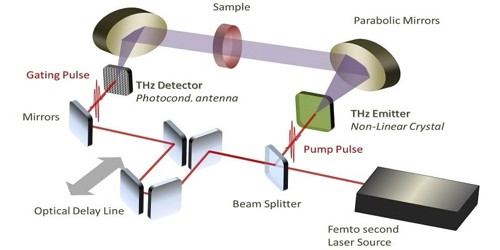Chemistry

# Time-domain SpectroscopyTime-domain spectroscopy is a type of spectroscopy that measures the power of electromagnetic radiation as a function of time. It is a tool for collecting spectral measurements in the far-infrared (approximately 3 – 100 wavenumbers). Normal spectroscopy is a frequency-domain spectroscopy. It is a powerful technique for material characterization and process control. It measures radiation as a function of frequency or wavelength. Time-domain spectroscopy usually requires the use of Fourier transforms. It is now a widely used tool to investigate materials parameters in the far-infrared spectral range (1 THz = 4 meV = 300 µm) without the need of using Kramers-Kronig transformations.

As the most common use of TDS is the reflectance mode, attention is given to reflectance measurement techniques and analysis, specifically the application of a numerical algorithm to reduce the effects of the quarter and half wavelength resonant. Absorptions in spectra usually look like broad peaks. This means that a peak may contain many wavelengths of radiation. These wavelengths can be plotted in the time domain. Notice that there are now smaller areas of constructive interference. This is because the closely spaced wavelengths quickly become more and more out of phase.

Applications

Time-domain spectroscopy is used very often in infrared spectroscopy (FT-IR) and NMR spectroscopy(FT-NMR). It is used to characterize material properties by measuring the complex frequency response within the frequency range of 0.1 THz to tens of THz. It has several advantages over frequency-domain spectroscopy. In frequency-domain experiments, each wavelength must be measured one at a time. This means scans can take several minutes. A single time-domain experiment takes no longer than a minute. Usually, it only takes seconds. This means that many scans may be taken for a given sample and that the scans can be averaged. So not only is the time-domain experiment faster, but it can also have a better signal to noise ratio.